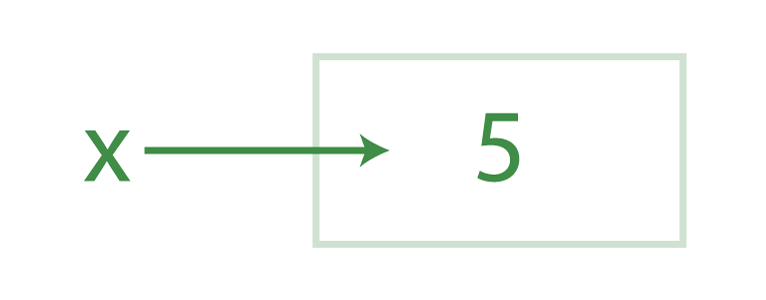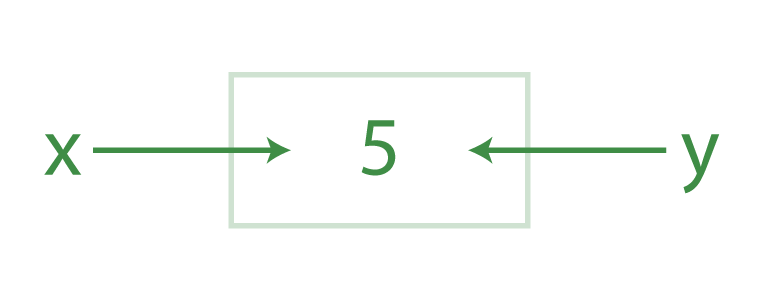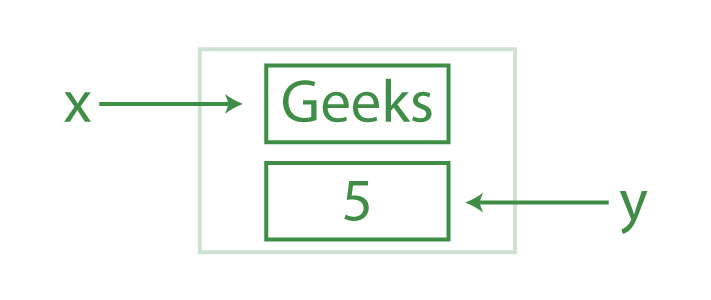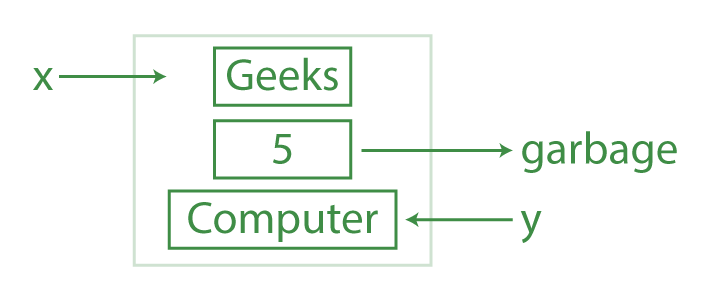GFG App
Open AppBrowser
Continue

Python Variable is containers that store values. Python is not “statically typed”. We do not need to declare variables before using them or declare their type. A variable is created the moment we first assign a value to it. A Python variable is a name given to a memory location. It is the basic unit of storage in a program.

## Example of Variable in Python

An Example of a Variable in Python is a representational name that serves as a pointer to an object. Once an object is assigned to a variable, it can be referred to by that name. In layman’s terms, we can say that Variable in Python is containers that store values.

Here we have stored “Geeksforgeeks”  in a var which is variable, and when we call its name the stored information will get printed.

## Python3

 `Var ``=` `"Geeksforgeeks"` `print``(Var)`

Output:

`Geeksforgeeks`

Notes:

• The value stored in a variable can be changed during program execution.
• A Variables in Python is only a name given to a memory location, all the operations done on the variable effects that memory location.

### Rules for Python variables

• A Python variable name must start with a letter or the underscore character.
• A Python variable name can only contain alpha-numeric characters and underscores (A-z, 0-9, and _ ).
• Variable in Python names are case-sensitive (name, Name, and NAME are three different variables).
• The reserved words(keywords) in Python cannot be used to name the variable in Python.

Example

## Python3

 `# valid variable name` `geeks ``=` `1` `Geeks ``=` `2` `Ge_e_ks ``=` `5` `_geeks ``=` `6` `geeks_ ``=` `7` `_GEEKS_ ``=` `8`   `print``(geeks, Geeks, Ge_e_ks)` `print``(_geeks, geeks_, _GEEKS_)`

Output:

```1 2 5
6 7 8```

## Variables Assignment in Python

Here, we have assigned a number, a floating point number, and a string to a variable such as age, salary, and name.

## Python3

 `# An integer assignment` `age ``=` `45`   `# A floating point` `salary ``=` `1456.8`   `# A string` `name ``=` `"John"`   `print``(age)` `print``(salary)` `print``(name)`

Output:

```45
1456.8
John```

### Declaration and Initialization of Variables

Let’s see how to declare the variable and print the variable.

## Python3

 `# declaring the var` `Number ``=` `100`   `# display` `print``( Number)`

Output:

`100`

### Redeclaring variables in Python

We can re-declare the Python variable once we have declared the variable already.

## Python3

 `# declaring the var` `Number ``=` `100`   `# display` `print``(``"Before declare: "``, Number)`   `# re-declare the var` `Number ``=` `120.3` `  `  `print``(``"After re-declare:"``, Number)`

Output:

```Before declare:  100
After re-declare: 120.3```

### Python Assign Values to Multiple Variables

Also, Python allows assigning a single value to several variables simultaneously with “=” operators.
For example:

## Python3

 `a ``=` `b ``=` `c ``=` `10`   `print``(a)` `print``(b)` `print``(c)`

Output:

```10
10
10```

### Assigning different values to multiple variables

Python allows adding different values in a single line with “,” operators.

## Python3

 `a, b, c ``=` `1``, ``20.2``, ``"GeeksforGeeks"`   `print``(a)` `print``(b)` `print``(c)`

Output:

```1
20.2
GeeksforGeeks```

### Can we use the same name for different types?

If we use the same name, the variable starts referring to a new value and type.

## Python3

 `a ``=` `10` `a ``=` `"GeeksforGeeks"`   `print``(a)`

Output:

`GeeksforGeeks`

### How does + operator work with variables?

The Python plus operator + provides a convenient way to add a value if it is a number and concatenate if it is a string. If a variable is already created it assigns the new value back to the same variable.

## Python3

 `a ``=` `10` `b ``=` `20` `print``(a``+``b)`   `a ``=` `"Geeksfor"` `b ``=` `"Geeks"` `print``(a``+``b)`

Output

```30
GeeksforGeeks```

### Can we use + for different Datatypes also?

No use for different types would produce an error.

## Python3

 `a ``=` `10` `b ``=` `"Geeks"` `print``(a``+``b)`

Output :

`TypeError: unsupported operand type(s) for +: 'int' and 'str'`

## Global and Local Python Variables

Local variables in Python are the ones that are defined and declared inside a function. We can not call this variable outside the function.

## Python3

 `# This function uses global variable s` `def` `f():` `    ``s ``=` `"Welcome geeks"` `    ``print``(s)`     `f()`

Output:

`Welcome geeks`

Global variables in Python are the ones that are defined and declared outside a function, and we need to use them inside a function.

## Python3

 `# This function has a variable with` `# name same as s.` `def` `f():` `    ``print``(s)`     `# Global scope` `s ``=` `"I love Geeksforgeeks"` `f()`

Output:

`I love Geeksforgeeks`

## Global keyword in Python

Python global is a keyword that allows a user to modify a variable outside of the current scope. It is used to create global variables from a non-global scope i.e inside a function. Global keyword is used inside a function only when we want to do assignments or when we want to change a variable. Global is not needed for printing and accessing.

### Rules of global keyword

• If a variable is assigned a value anywhere within the function’s body, it’s assumed to be local unless explicitly declared as global.
• Variables that are only referenced inside a function are implicitly global.
• We use a global in Python to use a global variable inside a function.
• There is no need to use a global keyword in Python outside a function.

Example:

Python program to modify a global value inside a function.

## Python3

 `x ``=` `15`   `def` `change():`   `    ``# using a global keyword` `    ``global` `x`   `    ``# increment value of a by 5` `    ``x ``=` `x ``+` `5` `    ``print``(``"Value of x inside a function :"``, x)`     `change()` `print``(``"Value of x outside a function :"``, x)`

Output:

```Value of x inside a function : 20
Value of x outside a function : 20```

## Variable Types in Python

Data types are the classification or categorization of data items. It represents the kind of value that tells what operations can be performed on a particular data. Since everything is an object in Python programming, data types are actually classes and variables are instances (object) of these classes.

### Built-in Python Data types are:

Example:

In this example, we have shown different examples of Built-in data types in Python.

## Python3

 `# numberic` `var ``=` `123` `print``(``"Numeric data : "``, var)`   `# Sequence Type` `String1 ``=` `'Welcome to the Geeks World'` `print``(``"String with the use of Single Quotes: "``)` `print``(String1)`   `# Boolean` `print``(``type``(``True``))` `print``(``type``(``False``))`   `# Creating a Set with` `# the use of a String` `set1 ``=` `set``(``"GeeksForGeeks"``)` `print``(``"\nSet with the use of String: "``)` `print``(set1)`   `# Creating a Dictionary` `# with Integer Keys` `Dict` `=` `{``1``: ``'Geeks'``, ``2``: ``'For'``, ``3``: ``'Geeks'``}` `print``(``"\nDictionary with the use of Integer Keys: "``)` `print``(``Dict``)`

Output:

```Numeric data :  123
String with the use of Single Quotes:
Welcome to the Geeks World
<class 'bool'>
<class 'bool'>

Set with the use of String:
{'r', 'G', 'e', 'k', 'o', 's', 'F'}

Dictionary with the use of Integer Keys:
{1: 'Geeks', 2: 'For', 3: 'Geeks'}```

## Object Reference in Python

Let us assign a variable x to value 5.

`x = 5`Another variable is y to the variable x.

`y = x`When Python looks at the first statement, what it does is that, first, it creates an object to represent the value 5. Then, it creates the variable x if it doesn’t exist and made it a reference to this new object 5. The second line causes Python to create the variable y, and it is not assigned with x, rather it is made to reference that object that x does. The net effect is that the variables x and y wind up referencing the same object. This situation, with multiple names referencing the same object, is called a Shared Reference in Python.
Now, if we write:

`x = 'Geeks'`

This statement makes a new object to represent ‘Geeks’ and makes x reference this new object.Now if we assign the new value in Y, then the previous object refers to the garbage values.

`y = "Computer"`### Creating objects (or variables of a class type)

Please refer to Class, Object, and Members for more details.

## Python3

 `# Python program to show that the variables with a value` `# assigned in class declaration, are class variables and` `# variables inside methods and constructors are instance` `# variables.`   `# Class for Computer Science Student`     `class` `CSStudent:`   `    ``# Class Variable` `    ``stream ``=` `'cse'`   `    ``# The init method or constructor` `    ``def` `__init__(``self``, roll):`   `        ``# Instance Variable` `        ``self``.roll ``=` `roll`     `# Objects of CSStudent class` `a ``=` `CSStudent(``101``)` `b ``=` `CSStudent(``102``)`   `print``(a.stream)  ``# prints "cse"` `print``(b.stream)  ``# prints "cse"` `print``(a.roll)    ``# prints 101`   `# Class variables can be accessed using class` `# name also` `print``(CSStudent.stream)  ``# prints "cse"`

Output

```cse
cse
101
cse```

My Personal Notes arrow_drop_up